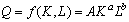# The Logarithmic Function

Linear functions are useful in economic models because a solution can easily be found.  However non-linear functions can be transformed into linear functions with the use of logarithms.

Given the functionTaking logarithms of both sides this function can be rewritten asThe resulting function is linear in the log of the variables.

### An application of the logarithmic function

A production function describes the relation between output and the inputs to a given process.  A simple production function might relate output to two inputs, labor and capital. (Capital typically includes plant and equipment.) A production function might be used to study tradeoffs in the use of capital and labor.

A well-known production function is the Cobb-Douglas Production function which has the formwhere

Q  =  the quantity of output

L  =  the quantity of labor

K  =  the quantity of capital

[Index]